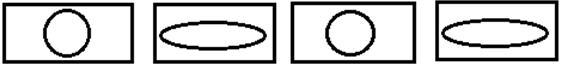Courses

# Test: Number - 1

## 10 Questions MCQ Test Mathematics for Class 1: NCERT | Test: Number - 1

Description
This mock test of Test: Number - 1 for Class 1 helps you for every Class 1 entrance exam. This contains 10 Multiple Choice Questions for Class 1 Test: Number - 1 (mcq) to study with solutions a complete question bank. The solved questions answers in this Test: Number - 1 quiz give you a good mix of easy questions and tough questions. Class 1 students definitely take this Test: Number - 1 exercise for a better result in the exam. You can find other Test: Number - 1 extra questions, long questions & short questions for Class 1 on EduRev as well by searching above.
QUESTION: 1

### Which sign shows that a number is equal to other numbers

Solution:

Equals to (=) sign is used to show that two numbers are equal to each other

like 2 = 2.

QUESTION: 2

Solution:
QUESTION: 3

### Which number is one less than 8 tens 4 ones?

Solution:
QUESTION: 4

Identify the odd one out.

Solution:
QUESTION: 5

Complete the following series.

Rs.11, Rs.12, Rs.13, Rs.14?

Solution:
QUESTION: 6

What is 7 tens and 2 ones?

Solution:
QUESTION: 7

Which of the following is the odd one in this series?

10, 30, 50, 70, 75, 90

Solution:
QUESTION: 8

Identify the next possible pattern and complete the series.Solution:
QUESTION: 9

Which of the following is the correct number name for 53?

Solution:
QUESTION: 10

Identify the odd one out in this series.

11, 12, 13, 14, 17

Solution: